# [Paper] Rethinking Cooperative Rationalization: Introspective Extraction and Complement Control

## Feb 14, 2021 · 831 words · 4 minute read nlp interpretability pytorch paperPhoto Credit

## Introduction

Model interpretability is crucial if we want to use AI models to make high-stake decisions (e.g., making medical diagnoses, preventing suicides, etc.). In NLP, one common way to get interpretability is to extract information from the trained models. For example, some use gradient-based input attribution techniques, some perturb the input to get explanations, and some use influence functions to find the most influential training examples to this particular input sequence. Another way is to make the model intrinsically explainable (e.g., a decision tree).

Selective rationalization creates self-explaining models without specialized designs nor architectural choices for the base model. This paper — “Rethinking Cooperative Rationalization: Introspective Extraction and Complement Control” — shows that we can get the self-explaining models with accuracy on par with the black-box ones with the proposed modification to the selective rationalization technique (i.e., introspective extraction and complement control).

## Selective Rationalization

The goal of selective rationalization is to extract only the portion of the text relevant for prediction. The original design consists of two players — a generator (that generates binary masks) and a predictor (that makes predictions based on the masked text).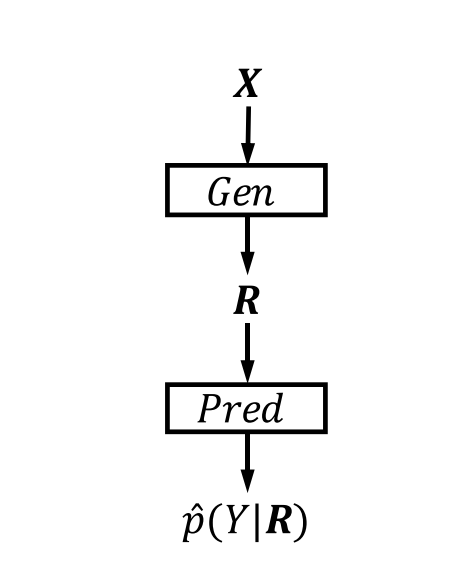The cooperative framework from . Source: 

Because the predictor can only see the extracted(masked) text, we can be 100% sure that the dropped tokens do not influence the prediction. (Note that we cannot be sure that 100% of the extracted text is relevant to the prediction, though. Nevertheless, we can increase the confidence by making the binary mask sparser.)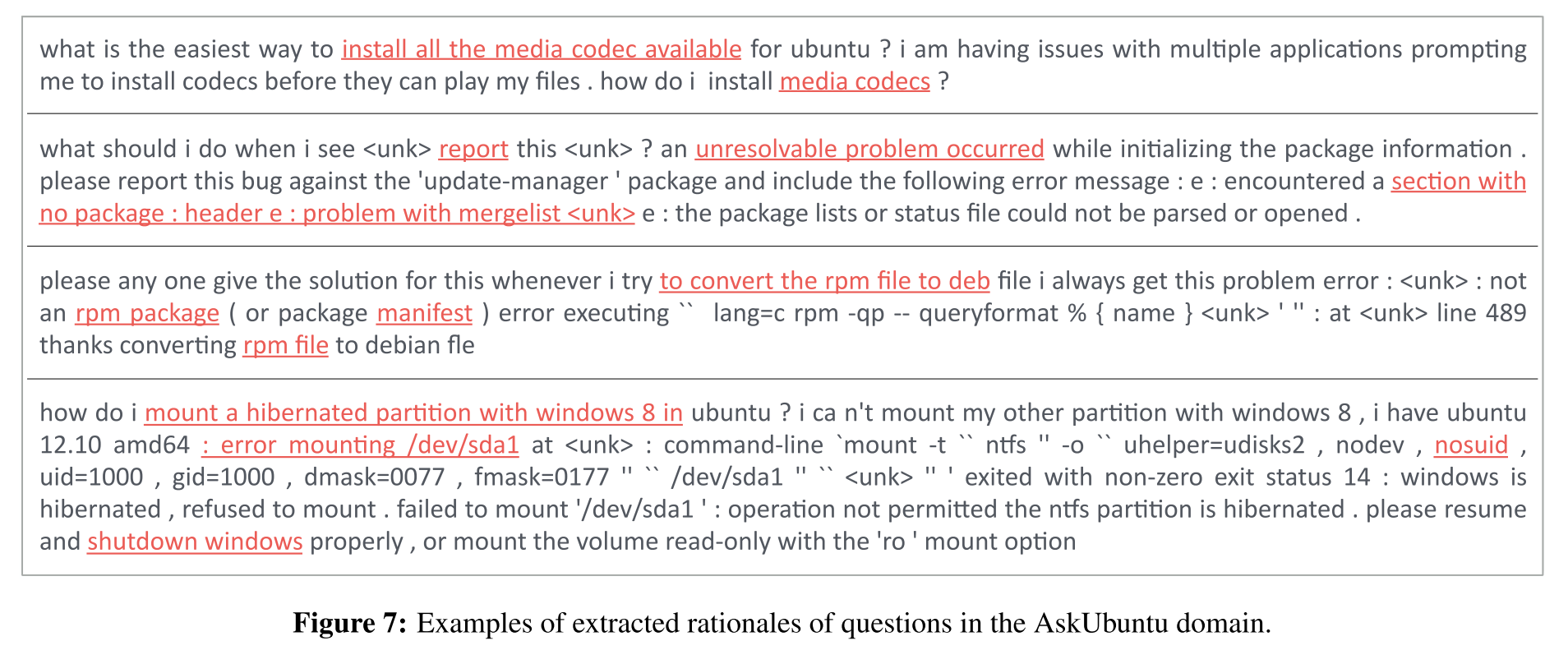Examples of extracted rationales of questions in the AskUbuntu domain. Source: 

## Complement Control

Degeneration refers to the situation where, rather than finding words in X that explain Y, R attempts to encode the probability of Y using trivial information, e.g., punctuation and position.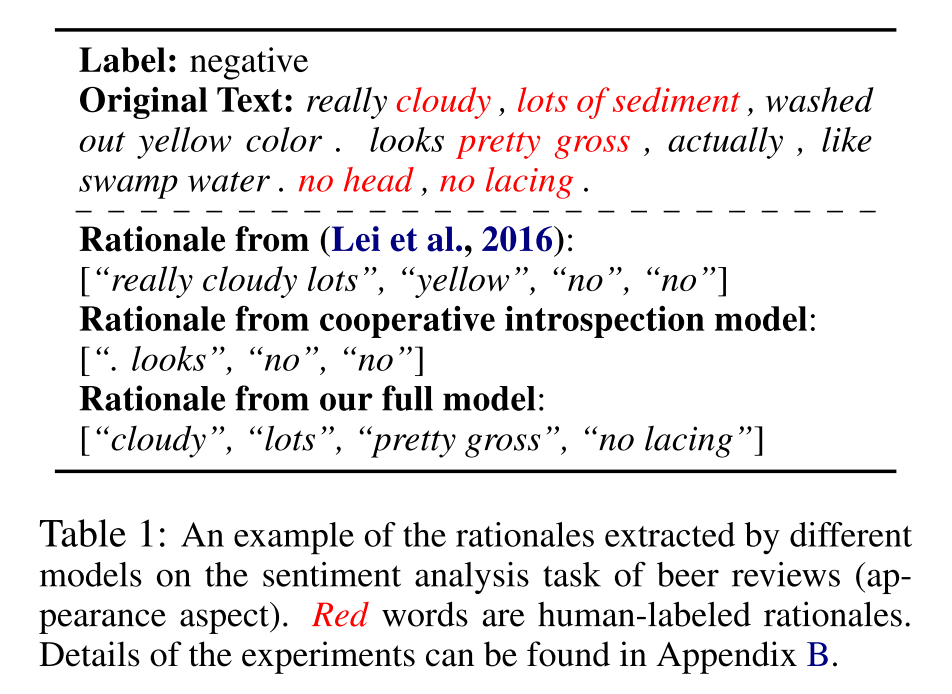An example of rationales extracted by different models. Source: 

To avoid degeneration (model taking shortcuts), the paper proposed that we train another predictor (the complement predictor) based on the dropped text. If we can still get decent accuracy from the complement predictor, we likely have degeneration at hand.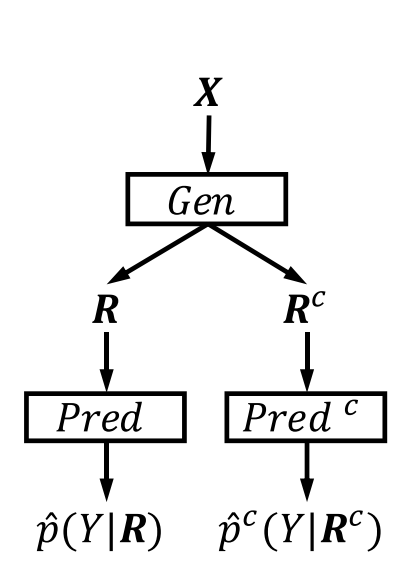Complement predictor.Source: 

The paper proposes that we penalize the model when the cross-entropy from the (actual) predictor is within h to the one from the complement predictor:

$$L_p = max\{L_p - L_c + h, 0\}$$

## Introspective Extraction

The paper posits out that since the generator in  typically has no direct access to the outcome it aims to justify, the learning process may converge to a poorly performing solution. The solution they proposed is to include the predicted class (from a pretrained model) in the input to the generator. In this scheme, the generator would know which label in advance the selected rationales will be used to predict.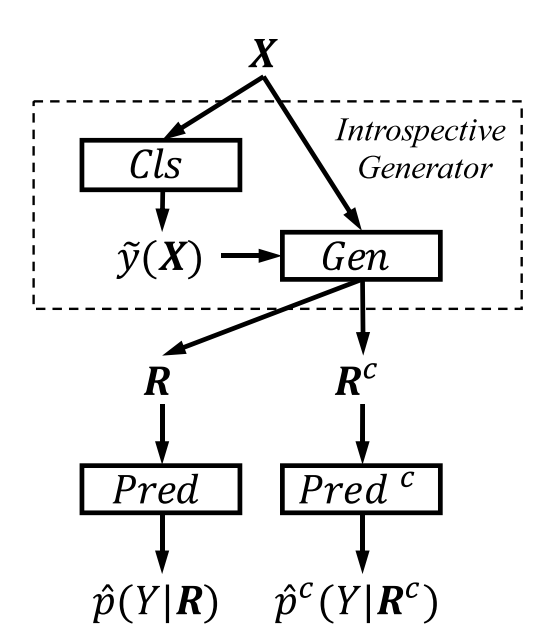Introspective generator.Source: 

(Notes from Ceshine) The reason for the emphasis on “no direct access” is that the generator actually has indirect access to the label via the cross-entropy of the predictor it tries to minimize (we’ll see more details in the next section). The generator has been implicitly predicting to which label this piece of text belongs. We can see the introspective extraction as a way to relieving the generator of this task and focus just on selecting the tokens.

## Training Generators

### Loss Function

This is the loss function for the generator:

$$\min_{z(\cdot)} L_p + \lambda_s L_g + \lambda_s L_s + \lambda_c L_c$$

We’ve covered $L_g$ in the Complement Control section. $L_s$ controls sparsity (penalizes the model when the number of the selected tokens exceeds a threshold), and $L_c$ controls continuity (penalizes the model when the number of gaps between selected tokens exceeds a threshold).

### Policy Gradient

Because the token mask is binary, we cannot directly use gradient descent to train the generator. Instead, the paper uses policy gradient to train the generator.

Policy gradient is commonly used in reinforcement learning. As the paper references , I think what they implement is the REINFORCE (Monte-Carlo policy gradient) algorithm. Since we have only one step in this design, it probably means sampling tokens according to the generated probabilities and taking averages.

(Notes from Ceshine) To have bounded rewards for training stability, the paper replace negative losses $L_p$ and $L_c$ with accuracy. I think clipped (negative) cross-entropy should have the same effect while supporting soft labels at the same time.

## Implementations

The paper provides an official implementation of the algorithm on Github. However, the code is a bit dated (it depends on PyTorch 0.3.0). I’ve found a modern implementation at interpretml/interpret-text, which also provides an example notebook.

Maybe I’ll write a part 2 that analyzes the implementation if I find it helpful enough. Stay tuned.

1. Yu, M., Chang, S., Zhang, Y., & Jaakkola, T. S. (2019). Rethinking cooperative rationalization: Introspective extraction and complement control.
2. Lei, T., Barzilay, R., & Jaakkola, T. (2016). Rationalizing neural predictions. EMNLP 2016 - Conference on Empirical Methods in Natural Language Processing, Proceedings, 107–117.
3. Willia, R. J. (1992). Simple Statistical Gradient-Following Algorithms for Connectionist Reinforcement Learning. Machine Learning, 8(3), 229–256.
4. Lilian Weng (2018). Policy Gradient Algorithms.
tweet Share